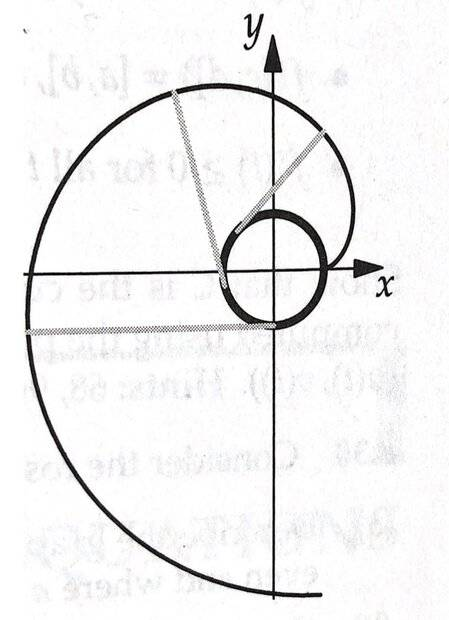# Finding parametric equations

i_love_science
Homework Statement:
Imagine a string (of negligible thickness) unwinding from a fixed circular bobbin of radius 10, so that the string unwound is always tangent to the bobbin, as in the picture attached. Assume that the string unwinds at the constant rate of one full loop of string per second. Determine parametric equations for the curve traced out by the end of the string, as shown by the dark curve in the picture.
Relevant Equations:
parametric equationsSolution:
The point of tangency of the string moves around the circle at ##2\pi## radians per second. First, we compute the position of the point of tangency of the string with the bobbin. Because this is simply a revolution around a circle of radius 10, the parameterization of the point of tangency is ##(10 \cos 2\pi t, 10 \sin 2\pi t)##. Now we compute the position of the end of the string relative to the point of tangency with the bobbin. After t seconds have passed, the point of tangency has moved a length of ##20\pi t## around the bobbin. Thus, a length of ##20\pi t## of string has been unwound. The direction that the string points is tangent to the bobbin. By computing the derivatives of the components in ##(10 \cos 2\pi t, 10 \sin 2\pi t)##, we realize that the direction the string points in is the direction of ##(\sin 2\pi t, – \cos 2\pi t)##.

I don't understand the bolded part above. Could anyone explain it? Thanks.

Last edited: